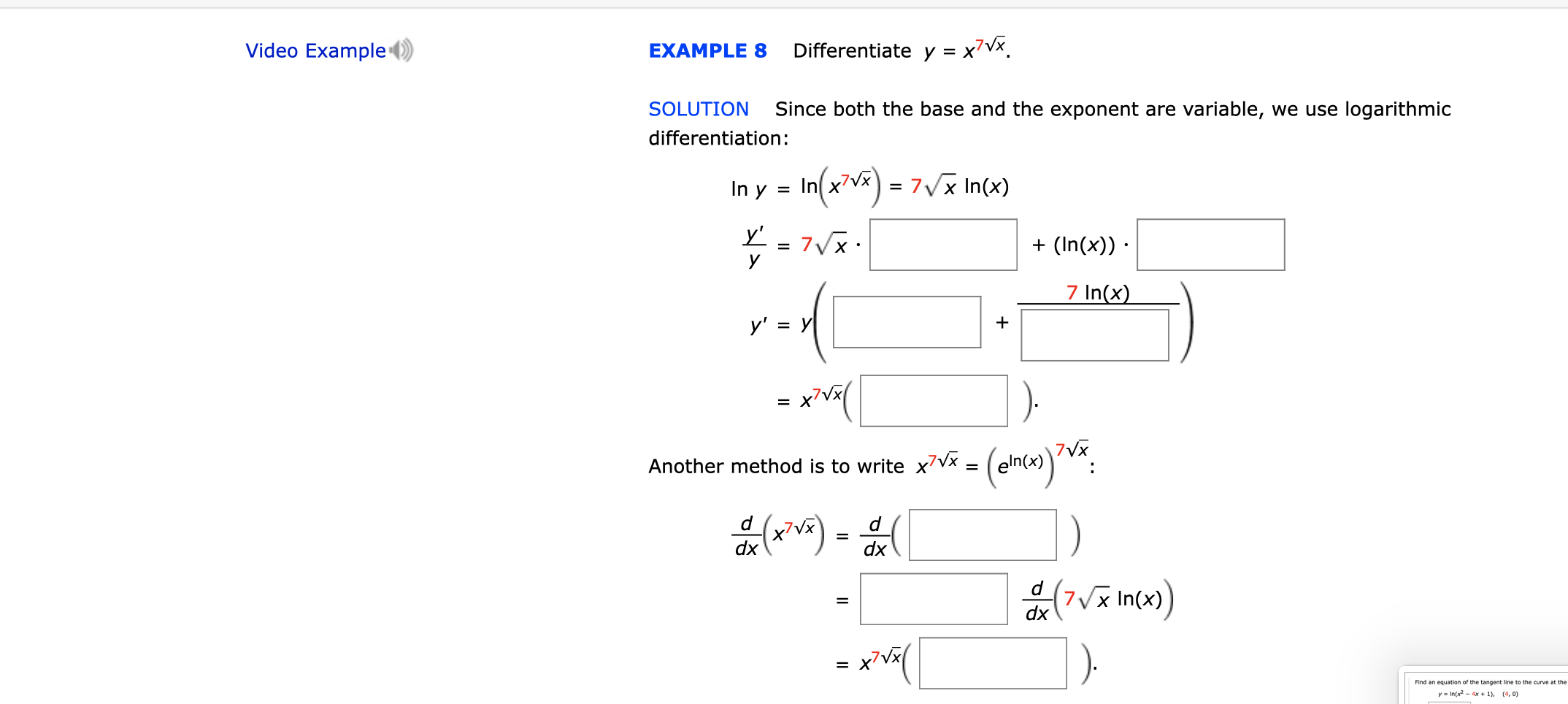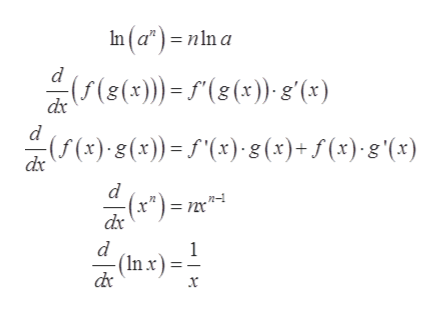# Video ExampleDifferentiate y = x7VxEXAMPLE 8Since both the base and the exponent are variable, we use logarithmicSOLUTIONdifferentiation:In(2)77Vx In(x)In y=y'7VXУ(In(x))7 In(x)у' %3D Уx'Vx7VXeln(x)Another method is to write x7Vx =dddxdxd7x In(x)dxx7Vx=yIn(x2-4x +1), (4, 0)

Question
3 viewshelp_outlineImage TranscriptioncloseVideo Example Differentiate y = x7Vx EXAMPLE 8 Since both the base and the exponent are variable, we use logarithmic SOLUTION differentiation: In(2)7 7Vx In(x) In y = y' 7VX У (In(x)) 7 In(x) у' %3D У x'Vx 7VX eln(x) Another method is to write x7Vx = d d dx dx d 7x In(x) dx x7Vx = yIn(x2-4x +1), (4, 0) fullscreen
check_circle

Step 1

To determine the logarithmic derivative and exponential derivative of the function.

Step 2

Given:

Step 3

Formula used:

...help_outlineImage TranscriptioncloseIn(a*)nln a (s())(8(x) g'(x) dx d (5 (x)-g(x))= 5'(x),g (x)+ f (x)g \(x) dx (x*)= nm* n dx d 1 (In x x fullscreen

### Want to see the full answer?

See Solution

#### Want to see this answer and more?

Solutions are written by subject experts who are available 24/7. Questions are typically answered within 1 hour.*

See Solution
*Response times may vary by subject and question.
Tagged in

### Derivative